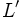# Derived subring of a Lie ring

(Redirected from Derived subring)
Let$L$ be a Lie ring. The derived subring or commutator subring of$L$, denoted$L'$, or$[L,L]$ is defined in the following ways:
• It is the additive subgroup generated by all elements of the form$[x,y]$, where$x,y \in L$
• It is the Lie subring generated by all elements of the form$[x,y]$, where$x,y \in L$
• It is the Lie ideal generated by all elements of the form$[x,y]$, where$x,y \in L$